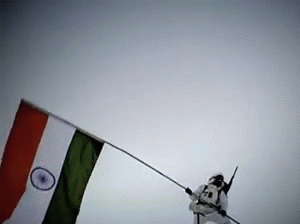### SBI PO 2015: Pie Chart - Data Interpretation

Dear Readers, We are providing with SBI PO 2015: Data Interpretation Question to solve for practice. Solve such questions and prepare for SBI PO 2015 exams

### SBI PO 2015: Pie Chart - Data Interpretation :

The following pie-chart shows the percentage distribution of the expenditure incurred in publishing a book. Study the pie-chart and the answer the questions based on it.

1.If for a certain quantity of books, the publisher has to pay Rs. 30,600 as printing cost, then what will be amount of royalty to be paid for these books?

A. Rs. 19,450 B. Rs. 21,200
C. Rs. 22,950 D. Rs. 26,150

2.What is the central angle of the sector corresponding to the expenditure incurred on Royalty?

A. 15º B. 24º
C. 54º D. 48º

3.The price of the book is marked 20% above the C.P. If the marked price of the book is Rs. 180, then what is the cost of the paper used in a single copy of the book?

A. Rs. 36 B. Rs. 37.50
C. Rs. 42 D. Rs. 44.25

4.Royalty on the book is less than the printing cost by:

A. 5% B.
33 1 %
5
C. 20% D. 25%

5.If 5500 copies are published and the transportation cost on them amounts to Rs. 82500, then what should be the selling price of the book so that the publisher can earn a profit of 25%?

A. Rs. 187.50 B. Rs. 191.50
C. Rs. 175 D. Rs. 180

6.For an edition of 12,500 copies, the amount of Royalty paid by the publisher is Rs. 2,81,250. What should be the selling price of the book if the publisher desires a profit of 5%?

A. Rs. 152.50 B. Rs. 157.50
C. Rs. 162.50 D. Rs. 167.50

7.If for an edition of the book, the cost of paper is Rs. 56250, then find the promotion cost for this edition.

A. Rs. 20,000 B. Rs. 22,500
C. Rs. 25,500 D. Rs. 28,125

8. Which two expenditures together have central angle of 108º?

A. Biding Cost and Transportation Cost
B. Printing Cost and Paper Cost
C. Royalty and Promotion Cost
D. Binding Cost and Paper Cost

For more such SBI PO related study materials visit us we would keep updating with SBI PO 2015 study materials. Also Like us on Facebook for updates.

wish you all the best!!!

## .## Proud to be an Indian